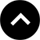Welcome to Tipsmate

Welcome to our one-stop destination for a myriad of calculators catering to diverse needs and interests. Whether you're navigating the intricacies of personal finance, embarking on a health and wellness journey, or in need of swift file conversions, our comprehensive collection of calculators is here to simplify your tasks. This page serves as a virtual toolkit, bringing together an array of specialized calculators designed to meet your specific requirements. From calculating mortgage payments to tracking your weight loss progress, and seamlessly converting files between formats, we've got you covered. Explore the world of precision and efficiency as you discover the calculators that make everyday tasks a breeze. Welcome to a realm where numbers meet convenience, making complex calculations feel like a walk in the park.

# Free Tools

#### Search By CategoryEnter various personal details and preferences. Then Clicks the "Calculate" button, it calculates and displays the estimated daily calorie intake for weight loss based on the provided information.Enter the initial weight and current weight. Then Click  the "Calculate" button, it calculates and displays the percentage of weight loss.Enter the loan amount, annual interest rate, and loan term in years. Then Click the "Calculate" button, it calculates and displays the estimated monthly VA loan payment.Enter the hourly wage, hours worked, and the tax rate as a percentage. Then Click the "Calculate" button, it calculates and displays the gross income, income tax deduction, and net income.Enter the rent amount, home price, annual interest rate, and other monthly costs. Then Click the "Calculate" button, it calculates and displays whether it may be financially better to rent or buy a home based on the estimated total costs.Enter the monthly rent amount, monthly utilities cost, and the number of roommates. Then Click the "Calculate" button, it calculates and displays the estimated monthly rent expenses per person.Enter the loan amount, annual interest rate, loan term in years, and the extra monthly payment. Then click the "Calculate" button, it calculates and displays the estimated time required to pay off the mortgage with the extra monthly payment.Enter the initial investment, annual contribution, annual interest rate, and investment duration in years. Then Click the "Calculate" button, it calculates and displays the estimated future value of the investment.Enter the principal amount, future value, and time period in years. Then Click the "Calculate" button, it calculates and displays the estimated annual interest rate.Enter the principal amount, annual interest rate, time period in years, and the type of interest (simple or compound). Then Click the "Calculate" button, it calculates and displays the interest amount and the total amount based on the chosen interest type.Enter the home price, down payment, annual interest rate, and loan term in years. Clicks the "Calculate" button, it calculates and displays the estimated monthly home loan payment.Enter the loan amount, annual interest rate, and loan term in years. Then Click the "Calculate" button, it calculates and displays the estimated monthly FHA loan payment, including upfront and monthly mortgage insurance premiums.Enter the home price and the desired down payment percentage. Then Click "Calculate" button, it calculates and displays the estimated down payment amount.Enter the monthly income and total monthly debt payments. Then Click the "Calculate" button, it calculates and displays the debt-to-income ratio as a percentage.Enter the nominal interest rate, loan fees, and loan term in years. When the user clicks the "Calculate" button, it calculates and displays the estimated APR of the loan.Enter the loan amount, annual interest rate, and loan term in years. Then Click the "Calculate" button, it calculates and displays the estimated monthly payment and generates an amortization table that shows the breakdown of each monthly payment into principal and interest.Enter car price, down payment, annual interest rate, and loan term in months. Clicks the "Calculate" button, and it will calculates and displays the estimated monthly car loan payment.Enter the principal amount, annual interest rate, time in years, and the number of times interest is compounded annually. Then Click the "Calculate" button, it calculates and display the compound interest.